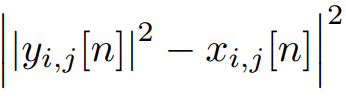# How to reformulate power of non-affine function in a CVX way

Hello, I aim to minimizewhere y is complex and x is given real, but when I use pow_pos( | |y|^2 - x |^2 , 2 ) or square_pos( | |y|^2 - x |^2 ), the solution will make |y|^2 - x < 0 and the result is very close to 0.

I found that maybe because
POW_POS(X,P) = POS(X).^P = MAX(X,0).^P,
SQUARE_POS(X)=MAX(X,0).^2,
but other power functions require X must be affine, how can I reformulate my objective function?

I really hope you can give me some suggestions.

This is not convex. see a special case (x^2-x)^2. so it cant be expressed by cvx.

1 Like

Very thanks for your help.